Function Repository Resource:

# MapReduceOperator

Like an operator form of GroupBy, but where one also specifies a reducer function to be applied

Contributed by: Seth J. Chandler
 ResourceFunction["MapReduceOperator"][f→g,h] creates an operator that will group its argument using function f, map g onto each top level element of its argument and then map the reducer function h over each grouping.

## Details and Options

ResourceFunction["MapReduceOperator"] takes the option "Parallelize". Its default value is False. If set to True, ParallelMap is used instead of Map.
The function works by using Curry to create a variant of GroupBy. It thus works similarly to Function[x,GroupBy[x,fg,h]].
The reducer function is referred to in the documentation for GroupBy as the combiner function.
ResourceFunction["MapReduceOperator"] accepts any functions in its arguments that GroupBy would accept.
ResourceFunction["MapReduceOperator"], like GroupBy, will not work where one wants successive groupings.
ParallelMap does not work on all functions. ResourceFunction["MapReduceOperator"] does not address this issue.

## Examples

### Basic Examples (2)

Group the data by the first element in each list, then get the last element of each list, and then compute the Mean of each grouping:

 In:=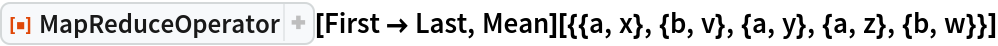Out=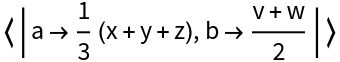Group a list of integers according to their parity, compute their square roots, and display the results as column vectors:

 In:=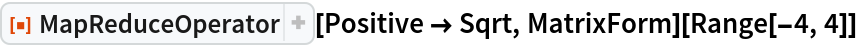Out=### Scope (2)

Use MapReduceOperator with slot functions to create an operator that works on lists of associations (optionally wrapped in Dataset) and then apply it to the Titanic dataset:

 In:=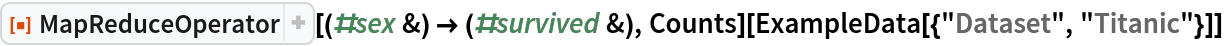Out=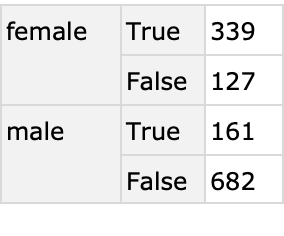The same query as above, but with the reducer function Counts run in parallel on the Normal form of the dataset:

 In:=Out=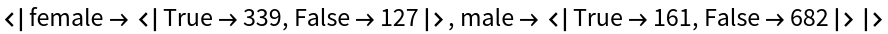### Options (2)

"Parallelize" can take on the value True or False. If it is set to anything else, it is treated as if it were False. "Parallelize" will produce error messages if the data is wrapped in Dataset:

 In:=Out=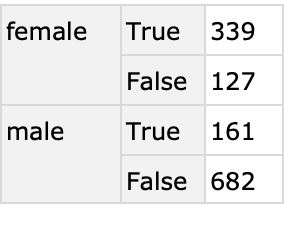Applying Normal to the dataset will fix this problem:

 In:=Out=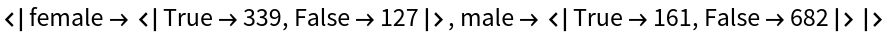### Applications (4)

Get histograms of the number of dates between the diagnosis of AIDS and death of the patient broken down by Australian state:

 In:=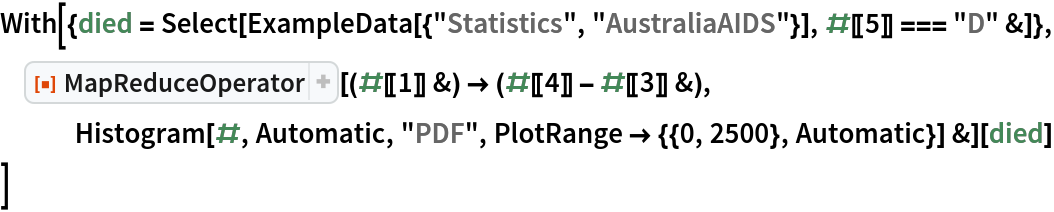Out=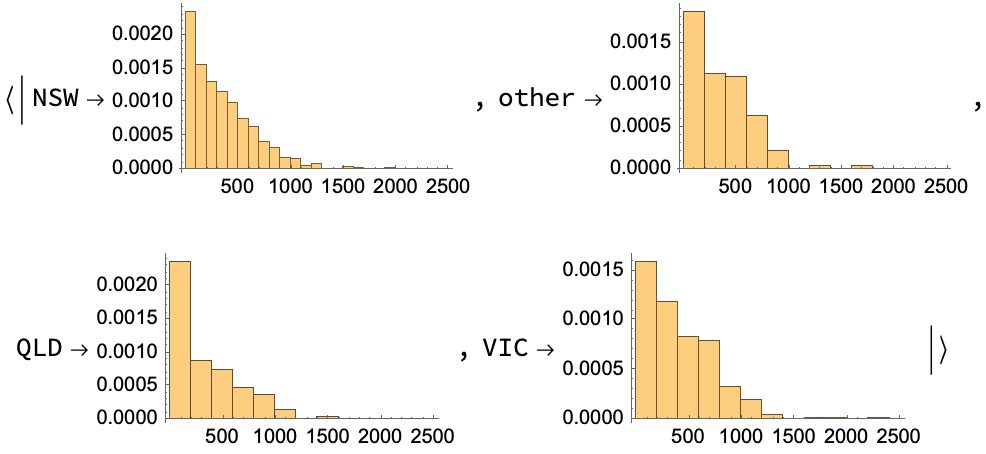Group the planets according to the number of moons and compute for each grouping the median volume of the planets (assuming they are spherical):

 In:=Out=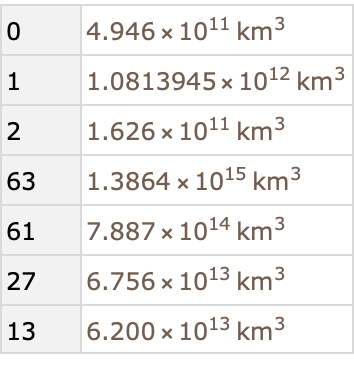Compute the fraction surviving (True) and dying on the Titanic as a function of their cabin class and sex and do so in parallel using a composition of the resource function Proportions and a built-in function:

 In:=Out=Compute the Mean age of passengers on the Titanic broken down by class and deleting data for which age is Missing:

 In:=Out=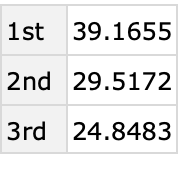### Possible Issues (1)

To omit the transformation of the grouped data, use Identity as follows:

 In:=Out=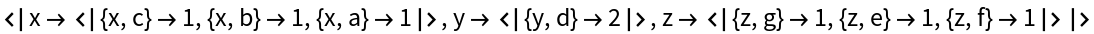Seth J. Chandler

## Version History

• 1.0.0 – 14 June 2019